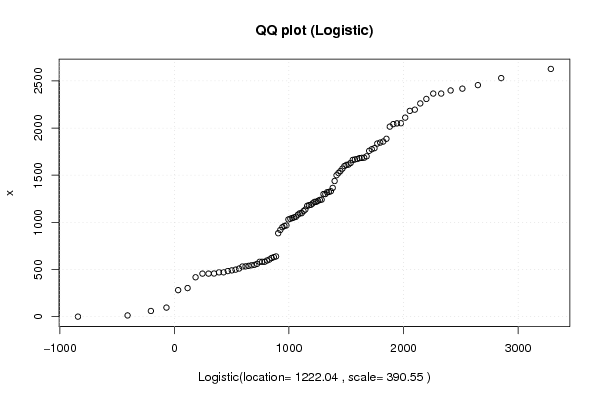## Free Statistics

of Irreproducible Research!

Author's title
Author*Unverified author*
R Software Modulerwasp_fitdistrlogistic.wasp
Title produced by softwareMaximum-likelihood Fitting - Logistic Distribution
Date of computationSun, 18 Apr 2010 10:04:15 +0000
Cite this page as followsStatistical Computations at FreeStatistics.org, Office for Research Development and Education, URL https://freestatistics.org/blog/index.php?v=date/2010/Apr/18/t12715851045auonje69ewg47g.htm/, Retrieved Tue, 28 Nov 2023 23:04:15 +0000
Statistical Computations at FreeStatistics.org, Office for Research Development and Education, URL https://freestatistics.org/blog/index.php?pk=74659, Retrieved Tue, 28 Nov 2023 23:04:15 +0000
QR Codes:Original text written by user:
IsPrivate?No (this computation is public)
User-defined keywordsFM55,steven,coomans,thesis,logistisch
Estimated Impact214
Family? (F = Feedback message, R = changed R code, M = changed R Module, P = changed Parameters, D = changed Data)
-       [Maximum-likelihood Fitting - Logistic Distribution] [FM55,steven,cooma...] [2010-04-18 10:04:15] [d41d8cd98f00b204e9800998ecf8427e] [Current]
Feedback Forum

Post a new message
Dataseries X:
13
96
60.1
281.25
548.59
470.325
456.25
456.25
483.5
498.25
457.5
490.7
303.075
510
417.7
631.4
595.625
531.15
581.725
581.725
544.75
582
622.225
638.666
469.9
532.78
538.5
884.65
559.08
1045.51
1319.34
0
1300
1322.6
962.57
1030.715
1038.31
967.87
1228.2
1300.285
1095.995
1181.955
1499.46
1523.38
1237.765
1119.545
1136.165
1081.63
1060.065
1216.67
1186.17
1217.475
1096.95
1685.6
1758.5
1786.6
2049.895
1845.895
2015.02
1609.63
918.725
1240.96
1671.785
1438.466
1886.14
2110.66
1856.87
1775.765
1569.625
1835.69
2041.46
1667.035
948.25
1365.66
1681.025
1661.9
2194.88
2051.025
2365.845
2398.5
2181.85
2626.77
2529.72
1700.3
605.38
1200.495
1597.02
1174.955
1612.88
1683.55
2260.955
2455.335
2365.62
2417.755
2308.785
1629.94
1053.275
1330.235
1543.85

 Summary of computational transaction Raw Input view raw input (R code) Raw Output view raw output of R engine Computing time 1 seconds R Server 'Sir Ronald Aylmer Fisher' @ 193.190.124.24

\begin{tabular}{lllllllll}
\hline
Summary of computational transaction \tabularnewline
Raw Input & view raw input (R code)  \tabularnewline
Raw Output & view raw output of R engine  \tabularnewline
Computing time & 1 seconds \tabularnewline
R Server & 'Sir Ronald Aylmer Fisher' @ 193.190.124.24 \tabularnewline
\hline
\end{tabular}
%Source: https://freestatistics.org/blog/index.php?pk=74659&T=0

[TABLE]
[ROW][C]Summary of computational transaction[/C][/ROW]
[ROW][C]Raw Input[/C][C]view raw input (R code) [/C][/ROW]
[ROW][C]Raw Output[/C][C]view raw output of R engine [/C][/ROW]
[ROW][C]Computing time[/C][C]1 seconds[/C][/ROW]
[ROW][C]R Server[/C][C]'Sir Ronald Aylmer Fisher' @ 193.190.124.24[/C][/ROW]
[/TABLE]
Source: https://freestatistics.org/blog/index.php?pk=74659&T=0

Globally Unique Identifier (entire table): ba.freestatistics.org/blog/index.php?pk=74659&T=0

As an alternative you can also use a QR Code:

The GUIDs for individual cells are displayed in the table below:

 Summary of computational transaction Raw Input view raw input (R code) Raw Output view raw output of R engine Computing time 1 seconds R Server 'Sir Ronald Aylmer Fisher' @ 193.190.124.24

 Parameter Estimated Value Standard Deviation location 1222.04275216191 69.5970309291708 scale 390.546140526781 31.9801482490995

\begin{tabular}{lllllllll}
\hline
Parameter & Estimated Value & Standard Deviation \tabularnewline
location & 1222.04275216191 & 69.5970309291708 \tabularnewline
scale & 390.546140526781 & 31.9801482490995 \tabularnewline
\hline
\end{tabular}
%Source: https://freestatistics.org/blog/index.php?pk=74659&T=1

[TABLE]
[ROW][C]Parameter[/C][C]Estimated Value[/C][C]Standard Deviation[/C][/ROW]
[ROW][C]location[/C][C]1222.04275216191[/C][C]69.5970309291708[/C][/ROW]
[ROW][C]scale[/C][C]390.546140526781[/C][C]31.9801482490995[/C][/ROW]
[/TABLE]
Source: https://freestatistics.org/blog/index.php?pk=74659&T=1

Globally Unique Identifier (entire table): ba.freestatistics.org/blog/index.php?pk=74659&T=1

As an alternative you can also use a QR Code:

The GUIDs for individual cells are displayed in the table below:

 Parameter Estimated Value Standard Deviation location 1222.04275216191 69.5970309291708 scale 390.546140526781 31.9801482490995PNG link Postscript link PDF link

Parameters (Session):
Parameters (R input):
R code (references can be found in the software module):
library(MASS)(f<-fitdistr(x, 'logistic'))xlab <- paste('Logistic(location=',round(f$estimate[],2))xlab <- paste(xlab,', scale=')xlab <- paste(xlab,round(f$estimate[],2))xlab <- paste(xlab,')')bitmap(file='test2.png')qqplot(qlogis(ppoints(x), location=f$estimate[], scale=f$estimate[]), x, main='QQ plot (Logistic)', xlab=xlab )grid()dev.off()load(file='createtable')a<-table.start()a<-table.row.start(a)a<-table.element(a,'Parameter',1,TRUE)a<-table.element(a,'Estimated Value',1,TRUE)a<-table.element(a,'Standard Deviation',1,TRUE)a<-table.row.end(a)a<-table.row.start(a)a<-table.element(a,'location',header=TRUE)a<-table.element(a,f$estimate)a<-table.element(a,f$sd)a<-table.row.end(a)a<-table.row.start(a)a<-table.element(a,'scale',header=TRUE)a<-table.element(a,f$estimate)a<-table.element(a,f$sd)a<-table.row.end(a)a<-table.end(a)table.save(a,file='mytable.tab')